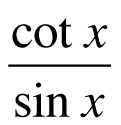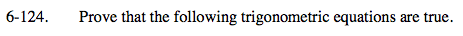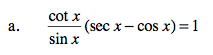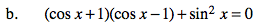### Home > PC > Chapter 6 > Lesson 6.3.2 > Problem6-124

6-124.
1. Prove that the following trigonometric equations are true. Homework Help ✎

1.(sec x − cos x) = 1

2. (cos x + 1)(cos x − 1) + sin2 x = 01. Change all terms to sine and cosine.
2. Get a common denominator for the terms in the parentheses.
3. Use the Fundamental Pythagorean Identity.1. Multiply out on the left side.
2. Use the Fundamental Pythagorean Identity.